Next: Conditional Constraints vs. Joint Up: CEM - A Maximum Previous: MAP Estimation

# Implementation and Interpretation

We now describe the implementation of the CEM algorithm as an iterative process. This typical time per iteration is comparable with a regular mixture of Gaussians model being update with EM. This is partly due to the decomposition of the variables into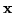and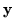. EM uses a large covariance matrix of both variables concatenated together and the matrix inversion in the EM-loop is thus of a higher dimensionality than the CEM matrix inversions of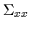and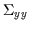. In high dimensions, therefore, when matrix inversions become a serious computational costs, the CEM algorithm can outperform EM in the per-iteration time. Some results will be shown in 2D problems that can be visually verified. Other higher dimensionality tests were also conducted and are reported as well.

Table 7.1: CEM Iteration Loop
 Start CE Step Compute the Conditional Expectation (the him and riparameters with respect to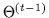M Step Update the Experts and the Mixing Proportions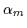CE Step Update the him and the ri due to the Change in the Experts and Mixing Proportions M Step Update the Gate Means CE Step Update the him and the ri due to the Change in the Gate Means M Step Update the Gate Covariances Repeat Replace Oldwith the New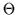and Return to Start

We assume that the conditional model is initialized using a pseudo-random method which places the initial Gaussians with some coverage of the data. From there the algorithm proceeds in a continuous loop as described by Table 7.1.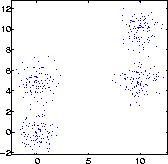Figure 7.7 depicts a set of 2D data points with coordinates (x,y). This data has been sampled from 4 Gaussians with circular covariance. The objective is to fit a conditional model p(y|x) to the above data with a conditioned mixture of Gaussians model with only 2 Gaussians. Thus, the algorithm can not simply group 4 clusters but must take advantage of some symmetric structure in the problem to find a more compact probabilistic model. This is thus a clear problem of limited resources where we would like to maximize performance at guessing output y from input x and we do not have the luxury of modeling the whole generative process (i.e. with 4 Gaussians). Thus, information about the particular task is critical here.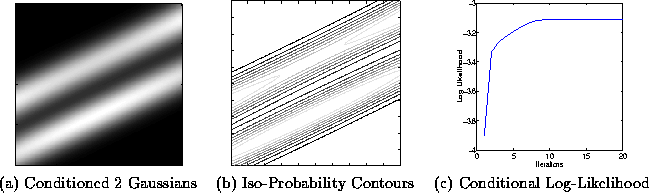In Figure 7.8 we note the maximum conditional likelihood solution which places the Gaussians in a skewed position to regress the y data given an x value. Note how the conditioned Gaussians give no information about x since the CEM expects it to be given. In Figure 7.9 we see another solution where the CEM was trapped in a slightly inferior local maximum (Conditional Log Likelihood = -3.166).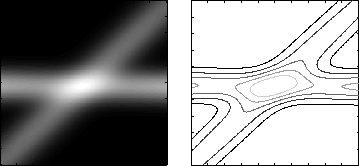Figure 7.10 shows the evolution of the EM algorithm on the exact same data set. We again plot the conditional log-likelihood and see that it is not being maximized (as expected) while the joint log-likelihood is being maximized. However, given that this is a conditional problem between x-input and y-output, the algorithm is not optimizing for the correct task and is not being discriminative. Thus the Gaussians are wasted modeling x and do not recover the bimodal distribution in y. The conditional log-likelihood is also dramatically inferior (-3.6).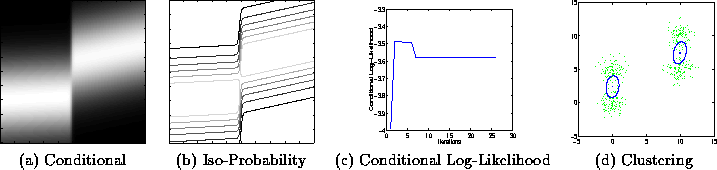The CEM algorithm, when initialized with a solution of the EM algorithm, typically moves to another solution. This solution is often better than the EM's solution but worse than initialization on its own. This indicates that the local maxima of joint likelihood are not necessarily maxima of conditional likelihood.Next: Conditional Constraints vs. Joint Up: CEM - A Maximum Previous: MAP Estimation
Tony Jebara
1999-09-15